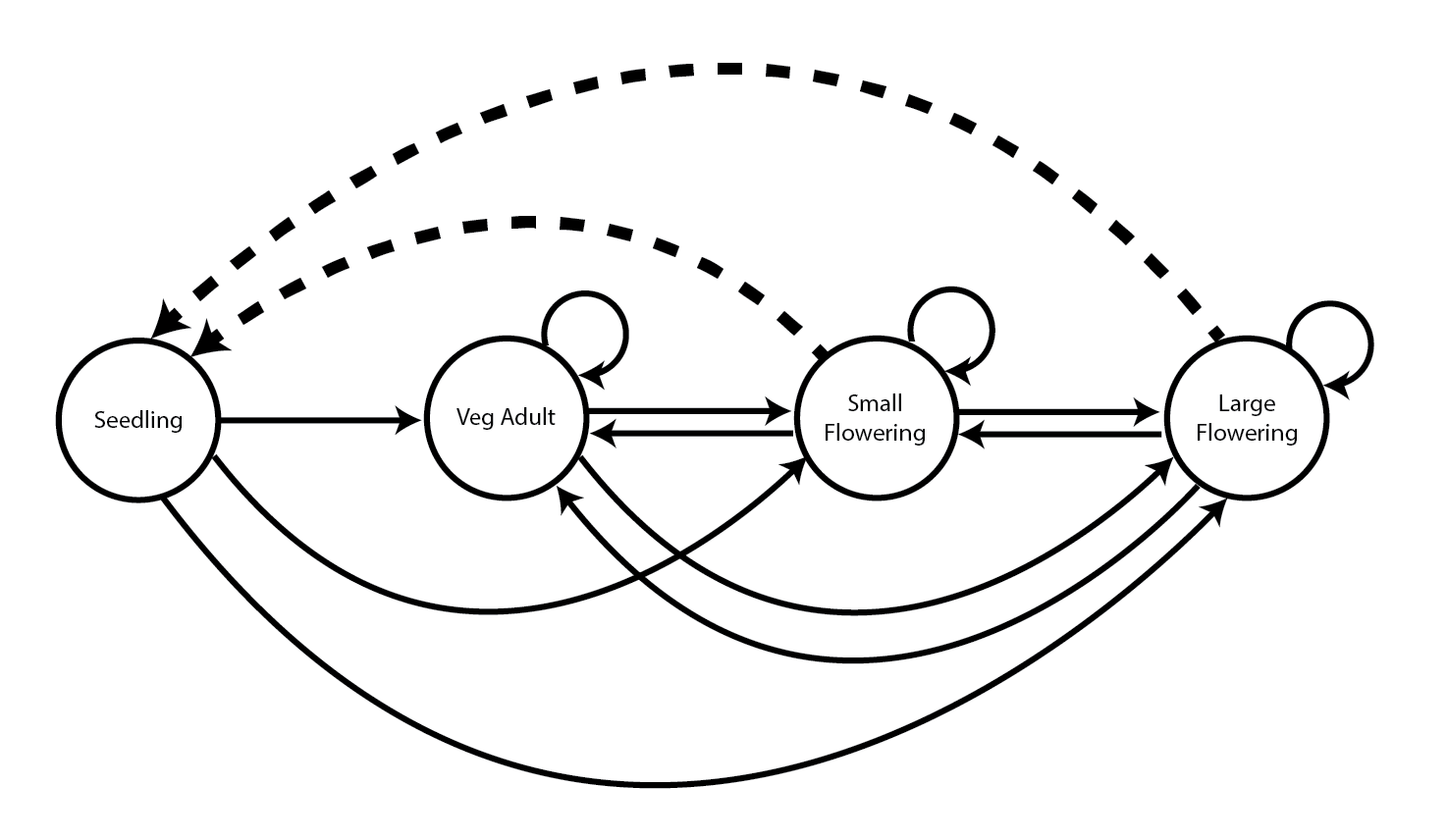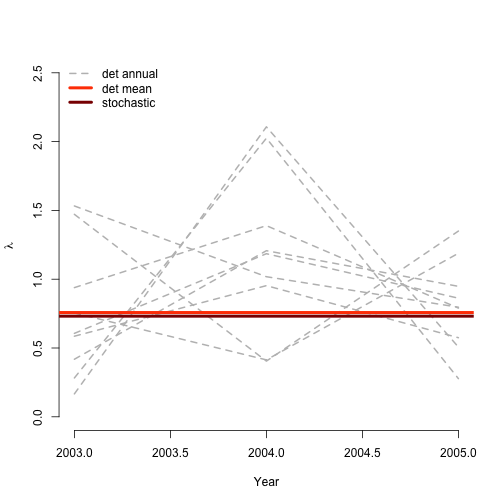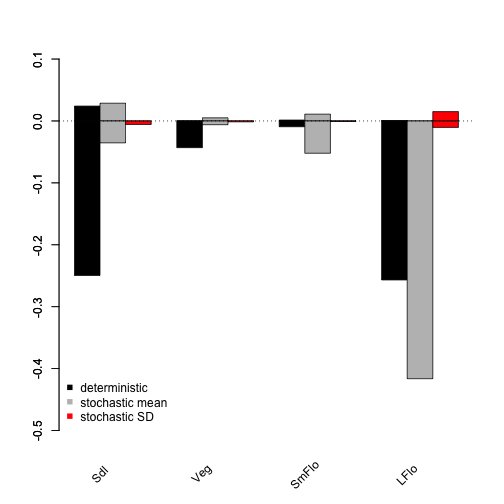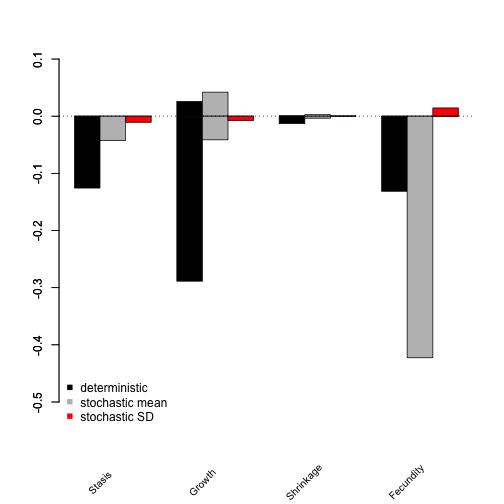# LTREs, sLTREs, and MPM import with Anthyllis vulneraria

In this vignette, we will import a MPM created elsewhere into lefko3 and analyze it via LTRE and sLTRE analysis. These are inherently ahistorical MPMs, and so we do not include historical analyses in this vignette. To reduce output size, we have prevented some statements from running if they produce long stretches of output. Examples include most summary() calls. In these cases, we include hashtagged versions of these calls, and our text assumes that the user runs these statements without hashtags. This vignette is only a sample analysis. Detailed information and instructions on using lefko3 are available through a free online e-book called lefko3: a gentle introduction, available on the projects page of the Shefferson lab website. This website also includes links to long-format vignettes and other demonstrations of this package.

### ORGANISM AND POPULATIONS

Davison et al. (2010) reported stochastic contributions made by differences in vital rate means and variances among nine natural populations of Anthyllis vulneraria, also known as kidney vetch, occurring in calcareous grasslands in the Viroin Valley of southwestern Belgium. A. vulneraria is a grassland specialist and the unique host plant of the Red-listed blue butterfly (Cupido minimus). It is a short-lived, rosette-forming legume with a complex life cycle including stasis and retrogression between four stages but no seedbank (seedlings, juveniles, small adults and large adults; Figure 9.1).Figure 9.1. Life history model of Anthyllis vulneraria. Solid arrows indicate survival transitions while dashed arrows indicate fecundity transitions.

Nine populations (N = 27-50,000) growing in distinct grassland fragments were surveyed between 2003 and 2006, yielding three (4x4) annual transition matrices for each population. The populations occurred within grassland fragments, and were mostly managed as nature reserves through rotational sheep grazing. These surveys coincided with a summer heat wave (2003), followed by a spring drought (2005) and an even more extreme heat wave (2006). These populations have been subject to detailed study for aspects of their genetics and demography, and further details on the sites can be obtained through the resulting publications (Krauss, Steffan-Dewenter, and Tscharntke 2004; Honnay et al. 2006; Piessens et al. 2009).

### BASIC WORKFLOW

Our goal in this exercise will be to import the published MPMs available for these nine populations of Anthyllis vulneraria, and to analyze the demographic differences between populations using deterministic and stochastic life table response experiments (LTRE and sLTRE). As we have the exact same matrices utilized in Davison et al. (2010), we will attempt to produce the exact same results as in that paper. We begin by loading package lefko3.

rm(list=ls(all=TRUE))
library(lefko3)

#### Step 1. Life history model development

We will first need to describe the life history characterizing the dataset, matching it to our analyses properly with a stageframe. Since we do not have the original demographic dataset that produced the published matrices, we do not need to know the exact sizes of plants and so will use proxy values. These proxy values need to be unique and need non-overlapping bins usable as size classes defining each stage. Other characteristics must be exact, however, to make sure that the analyses work properly. This includes all other stage descriptions, including reproductive status, propagule status, etc.

sizevector <- c(1, 1, 2, 3) # These sizes are not from the original paper
stagevector <- c("Sdl", "Veg", "SmFlo", "LFlo")
repvector <- c(0, 0, 1, 1)
obsvector <- c(1, 1, 1, 1)
matvector <- c(0, 1, 1, 1)
immvector <- c(1, 0, 0, 0)
propvector <- c(0, 0, 0, 0)
indataset <- c(1, 1, 1, 1)
binvec <- c(0.5, 0.5, 0.5, 0.5)
"Large flowering")

anthframe <- sf_create(sizes = sizevector, stagenames = stagevector,
repstatus = repvector, obsstatus = obsvector, matstatus = matvector,
immstatus = immvector, indataset = indataset, binhalfwidth = binvec,

#anthframe

#### Step 2. Data organization

Next we will enter the data for this vignette. Our data is in the form of matrices published in Davison et al. (2010). We will enter these matrices as standard R matrix class objects. All matrices are 4 rows by 4 columns big, and note that we fill them by row. As an example, let’s load the first matrix and take a look at it.

# POPN C 2003-2004
XC3 <- matrix(c(0, 0, 1.74, 1.74,
0.208333333, 0, 0, 0.057142857,
0.041666667, 0.076923077, 0, 0,
0.083333333, 0.076923077, 0.066666667, 0.028571429), 4, 4, byrow = TRUE)

XC3
#>            [,1]       [,2]       [,3]       [,4]
#> [1,] 0.00000000 0.00000000 1.74000000 1.74000000
#> [2,] 0.20833333 0.00000000 0.00000000 0.05714286
#> [3,] 0.04166667 0.07692308 0.00000000 0.00000000
#> [4,] 0.08333333 0.07692308 0.06666667 0.02857143

These are A matrices, meaning that they include all survival-transitions and fecundity for the population as a whole. The corresponding U and F matrices were not provided in that paper, although it is most likely that the elements valued at 1.74 in the top right-hand corner are only composed of fecundity values while the rest of the matrix is only composed of survival transitions (this might not be the case if clonal reproduction were possible). The order of rows and columns corresponds to the order of stages in the stageframe anthframe.

Let’s now load the remaining matrices.

# POPN C 2004-2005
XC4 <- matrix(c(0, 0, 0.3, 0.6,
0.32183908, 0.142857143, 0, 0,
0.16091954, 0.285714286, 0, 0,
0.252873563, 0.285714286, 0.5, 0.6), 4, 4, byrow = TRUE)

# POPN C 2005-2006
XC5 <- matrix(c(0, 0, 0.50625, 0.675,
0, 0, 0, 0.035714286,
0.1, 0.068965517, 0.0625, 0.107142857,
0.3, 0.137931034, 0, 0.071428571), 4, 4, byrow = TRUE)

# POPN E 2003-2004
XE3 <- matrix(c(0, 0, 2.44, 6.569230769,
0.196428571, 0, 0, 0,
0.125, 0.5, 0, 0,
0.160714286, 0.5, 0.133333333, 0.076923077), 4, 4, byrow = TRUE)

# POPN E 2004-2005
XE4 <- matrix(c(0, 0, 0.45, 0.646153846,
0.06557377, 0.090909091, 0.125, 0,
0.032786885, 0, 0.125, 0.076923077,
0.049180328, 0, 0.125, 0.230769231), 4, 4, byrow = TRUE)

# POPN E 2005-2006
XE5 <- matrix(c(0, 0, 2.85, 3.99,
0.083333333, 0, 0, 0,
0, 0, 0, 0,
0.416666667, 0.1, 0, 0.1), 4, 4, byrow = TRUE)

# POPN F 2003-2004
XF3 <- matrix(c(0, 0, 1.815, 7.058333333,
0.075949367, 0, 0.05, 0.083333333,
0.139240506, 0, 0, 0.25,
0.075949367, 0, 0, 0.083333333), 4, 4, byrow = TRUE)

# POPN F 2004-2005
XF4 <- matrix(c(0, 0, 1.233333333, 7.4,
0.223880597, 0, 0.111111111, 0.142857143,
0.134328358, 0.272727273, 0.166666667, 0.142857143,
0.119402985, 0.363636364, 0.055555556, 0.142857143), 4, 4, byrow = TRUE)

# POPN F 2005-2006
XF5 <- matrix(c(0, 0, 1.06, 3.372727273,
0.073170732, 0.025, 0.033333333, 0,
0.036585366, 0.15, 0.1, 0.136363636,
0.06097561, 0.225, 0.166666667, 0.272727273), 4, 4, byrow = TRUE)

# POPN G 2003-2004
XG3 <- matrix(c(0, 0, 0.245454545, 2.1,
0, 0, 0.045454545, 0,
0.125, 0, 0.090909091, 0,
0.125, 0, 0.090909091, 0.333333333), 4, 4, byrow = TRUE)

# POPN G 2004-2005
XG4 <- matrix(c(0, 0, 1.1, 1.54,
0.111111111, 0, 0, 0,
0, 0, 0, 0,
0.111111111, 0, 0, 0), 4, 4, byrow = TRUE)

# POPN G 2005-2006
XG5 <- matrix(c(0, 0, 0, 1.5,
0, 0, 0, 0,
0.090909091, 0, 0, 0,
0.545454545, 0.5, 0, 0.5), 4, 4, byrow = TRUE)

# POPN L 2003-2004
XL3 <- matrix(c(0, 0, 1.785365854, 1.856521739,
0.128571429, 0, 0, 0.010869565,
0.028571429, 0, 0, 0,
0.014285714, 0, 0, 0.02173913), 4, 4, byrow = TRUE)

# POPN L 2004-2005
XL4 <- matrix(c(0, 0, 14.25, 16.625,
0.131443299, 0.057142857, 0, 0.25,
0.144329897, 0, 0, 0,
0.092783505, 0.2, 0, 0.25), 4, 4, byrow = TRUE)

# POPN L 2005-2006
XL5 <- matrix(c(0, 0, 0.594642857, 1.765909091,
0, 0, 0.017857143, 0,
0.021052632, 0.018518519, 0.035714286, 0.045454545,
0.021052632, 0.018518519, 0.035714286, 0.068181818), 4, 4, byrow = TRUE)

# POPN O 2003-2004
XO3 <- matrix(c(0, 0, 11.5, 2.775862069,
0.6, 0.285714286, 0.333333333, 0.24137931,
0.04, 0.142857143, 0, 0,
0.16, 0.285714286, 0, 0.172413793), 4, 4, byrow = TRUE)

# POPN O 2004-2005
XO4 <- matrix(c(0, 0, 3.78, 1.225,
0.28358209, 0.171052632, 0, 0.166666667,
0.084577114, 0.026315789, 0, 0.055555556,
0.139303483, 0.447368421, 0, 0.305555556), 4, 4, byrow = TRUE)

# POPN O 2005-2006
XO5 <- matrix(c(0, 0, 1.542857143, 1.035616438,
0.126984127, 0.105263158, 0.047619048, 0.054794521,
0.095238095, 0.157894737, 0.19047619, 0.082191781,
0.111111111, 0.223684211, 0, 0.356164384), 4, 4, byrow = TRUE)

# POPN Q 2003-2004
XQ3 <- matrix(c(0, 0, 0.15, 0.175,
0, 0, 0, 0,
0, 0, 0, 0,
1, 0, 0, 0), 4, 4, byrow = TRUE)

# POPN Q 2004-2005
XQ4 <- matrix(c(0, 0, 0, 0.25,
0, 0, 0, 0,
0, 0, 0, 0,
1, 0.666666667, 0, 1), 4, 4, byrow = TRUE)

# POPN Q 2005-2006
XQ5 <- matrix(c(0, 0, 0, 1.428571429,
0, 0, 0, 0.142857143,
0.25, 0, 0, 0,
0.25, 0, 0, 0.571428571), 4, 4, byrow = TRUE)

# POPN R 2003-2004
XR3 <- matrix(c(0, 0, 0.7, 0.6125,
0.25, 0, 0, 0.125,
0, 0, 0, 0,
0.25, 0.166666667, 0, 0.25), 4, 4, byrow = TRUE)

# POPN R 2004-2005
XR4 <- matrix(c(0, 0, 0, 0.6,
0.285714286, 0, 0, 0,
0.285714286, 0.333333333, 0, 0,
0.285714286, 0.333333333, 0, 1), 4, 4, byrow = TRUE)

# POPN R 2005-2006
XR5 <- matrix(c(0, 0, 0.7, 0.6125,
0, 0, 0, 0,
0, 0, 0, 0,
0.333333333, 0, 0.333333333, 0.625), 4, 4, byrow = TRUE)

# POPN S 2003-2004
XS3 <- matrix(c(0, 0, 2.1, 0.816666667,
0.166666667, 0, 0, 0,
0, 0, 0, 0,
0, 0, 0, 0.166666667), 4, 4, byrow = TRUE)

# POPN S 2004-2005
XS4 <- matrix(c(0, 0, 0, 7,
0.333333333, 0.5, 0, 0,
0, 0, 0, 0,
0.333333333, 0, 0, 1), 4, 4, byrow = TRUE)

# POPN S 2005-2006
XS5 <- matrix(c(0, 0, 0, 1.4,
0, 0, 0, 0,
0, 0, 0, 0.2,
0.111111111, 0.75, 0, 0.2), 4, 4, byrow = TRUE)

We will incorporate all of these matrices into a lefkoMat object. To do so, we will organize the matrices in the proper order within a list. Feel free to remove the hashtag to see what the list looks like.

mats_list <- list(XC3, XC4, XC5, XE3, XE4, XE5, XF3, XF4, XF5, XG3, XG4, XG5,
XL3, XL4, XL5, XO3, XO4, XO5, XQ3, XQ4, XQ5, XR3, XR4, XR5, XS3, XS4, XS5)
#mats_list

Now we will create the lefkoMat object to hold these matrices. We will do this by calling the create_lM() function with the list object mats_list. We will also include metadata describing the order of populations (here treated as patches), and the order of monitoring occasions. Run the following lines, removing the hashtag to see what the object looks like.

anth_lefkoMat <- create_lM(mats_list, anthframe, hstages = NA,
historical = FALSE, poporder = 1,
patchorder = c(1, 1, 1, 2, 2, 2, 3, 3, 3, 4, 4, 4, 5, 5, 5, 6, 6, 6, 7, 7, 7,
8, 8, 8, 9, 9, 9),
yearorder = c(2003, 2004, 2005, 2003, 2004, 2005, 2003, 2004, 2005, 2003,
2004, 2005, 2003, 2004, 2005, 2003, 2004, 2005, 2003, 2004, 2005, 2003,
2004, 2005, 2003, 2004, 2005))
#anth_lefkoMat

The resulting object has all of the elements of a standard lefkoMat object except for those elements related to quality control in the demographic dataset and linear modeling. The option UFdecomp was left at its default (UFdecomp = TRUE), and so create_lM() used the stageframe to infer where fecundity values were located in the matrices and created U and F matrices separating those values. The default option for historical is set to FALSE, yielding an NA in places of the hstages element, which would typically list the order of historical stage pairs.

Let’s now take a look at a summary of our new lefkoMat object.

#summary(anth_lefkoMat)

The summary shows us that we have 27 matrices with 4 rows and columns each. The estimated numbers of transitions corresponds to the non-zero entries in each matrix. We see that we are covering 1 population, 9 patches, and 4 time steps. All of the survival probabilities observed fall within the bounds of 0 to 1, so everything seems alright.

#### Step 3. MPM analysis

Now, we will develop an arithmetic mean matrix and then assess the population growth rate.

anth_lmean <- lmean(anth_lefkoMat)

lambda2 <- lambda3(anth_lefkoMat)
lambda2m <- lambda3(anth_lmean)
set.seed(42)
sl2 <- slambda3(anth_lefkoMat) #Stochastic growth rate
sl2$expa <- exp(sl2$a)

plot(lambda ~ year2, data = subset(lambda2, patch == 1), ylim = c(0, 2.5),xlab = "Year",
ylab = expression(lambda), type = "l", col = "gray", lty= 2, lwd = 2, bty = "n")
lines(lambda ~ year2, data = subset(lambda2, patch == 2), col = "gray", lty= 2, lwd = 2)
lines(lambda ~ year2, data = subset(lambda2, patch == 3), col = "gray", lty= 2, lwd = 2)
lines(lambda ~ year2, data = subset(lambda2, patch == 4), col = "gray", lty= 2, lwd = 2)
lines(lambda ~ year2, data = subset(lambda2, patch == 5), col = "gray", lty= 2, lwd = 2)
lines(lambda ~ year2, data = subset(lambda2, patch == 6), col = "gray", lty= 2, lwd = 2)
lines(lambda ~ year2, data = subset(lambda2, patch == 7), col = "gray", lty= 2, lwd = 2)
lines(lambda ~ year2, data = subset(lambda2, patch == 8), col = "gray", lty= 2, lwd = 2)
lines(lambda ~ year2, data = subset(lambda2, patch == 9), col = "gray", lty= 2, lwd = 2)
abline(a = lambda2m$lambda, b = 0, lty = 1, lwd = 4, col = "orangered") abline(a = sl2$expa, b = 0, lty = 1, lwd = 4, col = "darkred")
legend("topleft", c("det annual", "det mean", "stochastic"), lty = c(2, 1, 1),
col = c("gray", "orangered", "darkred"), lwd = c(2, 4, 4), bty = "n")Figure 9.2. Deterministic vs. stochastic lambda

Very clearly, these populations exhibit extremely variable growth across the short interval of time that they were monitored. Also very clear is that they appear to be on the decline, as shown by $$\lambda$$ for the overall mean matrix, and the stochastic growth rate $$a = \text{log} \lambda$$.

At this point, let’s conduct a life table response experiment (LTRE). This will be a deterministic LTRE, meaning that we will assess the impacts of differences in matrix elements between the core matrices input via the lefkoMat object anth_lefkoMat and the overall arithmetic grand mean matrix. Note that we could use a different reference matrix if we wished, but the default if refmats = NA is to use the arithmetic grand mean. Please remove the hashtag from the end line of the next block to see the resulting output.

trialltre_det <- ltre3(anth_lefkoMat, refmats = NA, stochastic = FALSE,
steps = 10000, time_weights = NA, sparse = "auto")
#> Warning: Matrices input as mats will also be used as reference matrices.
#> Using all refmats matrices as reference matrices.
#trialltre_det

The resulting lefkoLTRE object gives the LTRE contributions for each matrix, including the annual matrices. While the differences in LTRE contributions across space are of interest, we cannot really infer differences across time this way because, across time, matrices are not related additively. To assess the contributions across space while accounting for temporal shifts, we should conduct a stochastic LTRE (sLTRE), as below.

trialltre_sto <- ltre3(anth_lefkoMat, refmats = NA, stochastic = TRUE,
steps = 10000, time_weights = NA, sparse = "auto")
#> Warning: Matrices input as mats will also be used as reference matrices.
#> Using all refmats matrices as reference matrices.
#trialltre_sto

The sLTRE produces output that is a bit different from the deterministic LTRE output. In the output, we see two lists of matrices prior to the MPM metadata. The first, ltre_mean, is a list of matrices showing the impact of differences in mean elements between the patch-level temporal mean matrices and the reference temporal mean matrix. The second, ltre_sd, is a list of matrices showing the impact of differences in the temporal standard deviation of each element between the patch-level and reference matrix sets. In other words, while a standard LTRE shows the impact of changes in matrix elements on $$\lambda$$, the sLTRE shows the impacts of changes in the mean and the variability of matrix elements on $$\text{log} \lambda$$. The labels element shows the order of matrices with reference to the populations or patches (remember that here, the populations are referred to as patches).

The output objects are large and can take a great deal of effort to look over and understand. Therefore, we will show three approaches to assessing these objects, using an approach similar to that used to assess elasticities. These methods can be used to assess patterns in all 9 populations, but for brevity we will focus only on the first population here. First, we will identify the elements most strongly impacting the population growth rate in each case.

writeLines(paste0("Highest (i.e most positive) deterministic LTRE contribution: ",
max(trialltre_det$ltre_det[]))) #> Highest (i.e most positive) deterministic LTRE contribution: 0.0240394864327486 writeLines(paste0("Highest deterministic LTRE contribution is associated with element: ", which(trialltre_det$ltre_det[] == max(trialltre_det$ltre_det[])))) #> Highest deterministic LTRE contribution is associated with element: 2 writeLines(paste0("Lowest (i.e. most negative) deterministic LTRE contribution: ", min(trialltre_det$ltre_det[])))
#> Lowest (i.e. most negative) deterministic LTRE contribution: -0.219474383631409
writeLines(paste0("Lowest deterministic LTRE contribution is associated with element: ",
which(trialltre_det$ltre_det[] == min(trialltre_det$ltre_det[]))))
#> Lowest deterministic LTRE contribution is associated with element: 4

writeLines(paste0("Highest stochastic mean LTRE contribution: ",
max(trialltre_sto$ltre_mean[]))) #> Highest stochastic mean LTRE contribution: 0.0179472041741181 writeLines(paste0("Highest stochastic mean LTRE contribution is associated with element: ", which(trialltre_sto$ltre_mean[] == max(trialltre_sto$ltre_mean[])))) #> Highest stochastic mean LTRE contribution is associated with element: 3 writeLines(paste0("Lowest stochastic mean LTRE contribution: ", min(trialltre_sto$ltre_mean[])))
#> Lowest stochastic mean LTRE contribution: -0.370937926897558
writeLines(paste0("Lowest stochastic mean LTRE contribution is associated with element: ",
which(trialltre_sto$ltre_mean[] == min(trialltre_sto$ltre_mean[]))))
#> Lowest stochastic mean LTRE contribution is associated with element: 13

writeLines(paste0("Highest stochastic SD LTRE contribution: ",
max(trialltre_sto$ltre_sd[]))) #> Highest stochastic SD LTRE contribution: 0.014441782311314 writeLines(paste0("Highest stochastic SD LTRE contribution is associated with element: ", which(trialltre_sto$ltre_sd[] == max(trialltre_sto$ltre_sd[])))) #> Highest stochastic SD LTRE contribution is associated with element: 13 writeLines(paste0("Lowest stochastic SD LTRE contribution: ", min(trialltre_sto$ltre_sd[])))
#> Lowest stochastic SD LTRE contribution: -0.0103367392514045
writeLines(paste0("Lowest stochastic SD LTRE contribution is associated with element: ",
which(trialltre_sto$ltre_sd[] == min(trialltre_sto$ltre_sd[]))))
#> Lowest stochastic SD LTRE contribution is associated with element: 16

writeLines(paste0("Total positive deterministic LTRE contributions: ",
sum(trialltre_det$ltre_det[][which(trialltre_det$ltre_det[] > 0)])))
#> Total positive deterministic LTRE contributions: 0.0267527462931098
writeLines(paste0("Total negative deterministic LTRE contributions: ",
sum(trialltre_det$ltre_det[][which(trialltre_det$ltre_det[] < 0)])))
#> Total negative deterministic LTRE contributions: -0.558699941273924
writeLines(paste0("Total positive stochastic mean LTRE contributions: ",
sum(trialltre_sto$ltre_mean[][which(trialltre_sto$ltre_mean[] > 0)])))
#> Total positive stochastic mean LTRE contributions: 0.0447245785886143
writeLines(paste0("Total negative stochastic mean LTRE contributions: ",
sum(trialltre_sto$ltre_mean[][which(trialltre_sto$ltre_mean[] < 0)])))
#> Total negative stochastic mean LTRE contributions: -0.509757776771075
writeLines(paste0("Total positive stochastic SD LTRE contributions: ",
sum(trialltre_sto$ltre_sd[][which(trialltre_sto$ltre_sd[] > 0)])))
#> Total positive stochastic SD LTRE contributions: 0.0151419474124082
writeLines(paste0("Total negative stochastic SD LTRE contributions: ",
sum(trialltre_sto$ltre_sd[][which(trialltre_sto$ltre_sd[] < 0)])))
#> Total negative stochastic SD LTRE contributions: -0.018346643772654

The output for the deterministic LTRE shows that element 4, which is the growth transition from seedling to large flowering adult (column 1, row 4), has the strongest influence. This contribution is negative, so it reduces $$\lambda$$. The most positive contribution to $$\lambda$$ is from element 2, which is the growth transition from seedling to vegetative adult (column 1, row 2). We also see the most positive contribution from shifts in mean elements in the sLTRE is element 3 (transition from seedling to small flowering adult), but the overall greatest impact is a negative contribution from element 13, which is the fecundity transition from large flowering adult to seedling (column 4, row 1). Variability in elements also contributes to shifts in $$\text{log} \lambda$$, though less so than shifts in mean elements. The strongest positive contribution is from variation in the fecundity transition from large flowering adult to seedling (column 4, row 1), while the most negative contribution is from stasis as a large flowering adult (row and column 4).

Second, we will identify which age-stages exert the strongest impact on the population growth rate.

ltre_pos <- trialltre_det$ltre_det[] ltre_neg <- trialltre_det$ltre_det[]
ltre_pos[which(ltre_pos < 0)] <- 0
ltre_neg[which(ltre_neg > 0)] <- 0

sltre_meanpos <- trialltre_sto$ltre_mean[] sltre_meanneg <- trialltre_sto$ltre_mean[]
sltre_meanpos[which(sltre_meanpos < 0)] <- 0
sltre_meanneg[which(sltre_meanneg > 0)] <- 0

sltre_sdpos <- trialltre_sto$ltre_sd[] sltre_sdneg <- trialltre_sto$ltre_sd[]
sltre_sdpos[which(sltre_sdpos < 0)] <- 0
sltre_sdneg[which(sltre_sdneg > 0)] <- 0

ltresums_pos <- cbind(colSums(ltre_pos), colSums(sltre_meanpos), colSums(sltre_sdpos))
ltresums_neg <- cbind(colSums(ltre_neg), colSums(sltre_meanneg), colSums(sltre_sdneg))

ltre_as_names <- trialltre_det$ahstages$stage

barplot(t(ltresums_pos), beside = T, col = c("black", "grey", "red"),
ylim = c(-0.50, 0.10))
barplot(t(ltresums_neg), beside = T, col = c("black", "grey", "red"), add = TRUE)
abline(0, 0, lty= 3)
text(cex=1, y = -0.57, x = seq(from = 2, to = 3.98*length(ltre_as_names),
by = 4), ltre_as_names, xpd=TRUE, srt=45)
legend("bottomleft", c("deterministic", "stochastic mean", "stochastic SD"),
col = c("black", "grey", "red"), pch = 15, bty = "n")Figure 9.3. LTRE contributions by age-stage

The output above shows that large flowering adults exert the strongest influence on both $$\lambda$$ and $$\text{log} \lambda$$, with the latter influence being through the impact of shifts in the mean. This impact is overwhelmingly negative. The next largest impact comes from seedlings in the deterministic case, and from small flowering adults in the stochastic case, in both cases the influence being negative on the whole.

Finally, we will assess what transition types exert the greatest impact on population growth rate.

det_ltre_summary <- summary(trialltre_det)
sto_ltre_summary <- summary(trialltre_sto)

ltresums_tpos <- cbind(det_ltre_summary$ahist_det$matrix1_pos,
sto_ltre_summary$ahist_mean$matrix1_pos,
sto_ltre_summary$ahist_sd$matrix1_pos)
ltresums_tneg <- cbind(det_ltre_summary$ahist_det$matrix1_neg,
sto_ltre_summary$ahist_mean$matrix1_neg,
sto_ltre_summary$ahist_sd$matrix1_neg)

barplot(t(ltresums_tpos), beside = T, col = c("black", "grey", "red"),
ylim = c(-0.55, 0.10))
barplot(t(ltresums_tneg), beside = T, col = c("black", "grey", "red"),
abline(0, 0, lty = 3)
text(cex=0.85, y = -0.64, x = seq(from = 2, to = 3.98*length(det_ltre_summary$ahist_det$category),
by = 4), det_ltre_summary$ahist_det$category, xpd=TRUE, srt=45)
legend("bottomleft", c("deterministic", "stochastic mean", "stochastic SD"),
col = c("black", "grey", "red"), pch = 15, bty = "n")Figure 9.4. LTRE contributions by transition type

The overall greatest impact on the population growth rate is from growth transitions in the deterministic case, but from fecundity transitions in the stochastic case. Clearly temporal variation has strong effects here that deserve to be assessed properly.

LTREs and sLTREs are powerful tools, and more complex versions of both analyses exist. Please consult Caswell (2001) and Davison et al. (2013) for more information.

## Literature cited

Caswell, Hal. 2001. Matrix Population Models: Construction, Analysis, and Interpretation. Second edition. Sunderland, Massachusetts, USA: Sinauer Associates, Inc.
Davison, Raziel, Hans Jacquemyn, Dries Adriaens, Olivier Honnay, Hans de Kroon, and Shripad Tuljapurkar. 2010. “Demographic Effects of Extreme Weather Events on a Short-Lived Calcareous Grassland Species: Stochastic Life Table Response Experiments.” Journal of Ecology 98 (2): 255–67. https://doi.org/10.1111/j.1365-2745.2009.01611.x.
Davison, Raziel, Florence Nicole, Hans Jacquemyn, and Shripad Tuljapurkar. 2013. “Contributions of Covariance: Decomposing the Components of Stochastic Population Growth in Cypripedium Calceolus.” The American Naturalist 181 (3): 410–20. https://doi.org/10.1086/669155.
Honnay, Olivier, Els Coart, Jan Butaye, Dries Adriaens, Sabine van Glabeke, and Isabel Roldan-Ruiz. 2006. “Low Impact of Present and Historical Landscape Configuration on the Genetics of Fragmented Anthyllis Vulneraria Populations.” Biological Conservation 127 (4): 411–19. https://doi.org/10.1016/j.biocon.2005.09.006.
Krauss, Jochen, Ingolf Steffan-Dewenter, and Tscharntke. 2004. “Landscape Occupancy and Local Population Size Depends on Host Plant Distribution in the Butterfly Cupido Minimus.” Biological Conservation 120 (3): 355–61. https://doi.org/10.1016/j.biocon.2004.03.007.
Piessens, Katrien, Dries Adriaens, Hans Jacquemyn, and Olivier Honnay. 2009. “Synergistic Effects of an Extreme Weather Event and Habitat Fragmentation on a Specialised Insect Herbivore.” Oecologia 159 (1): 117–26. https://doi.org/10.1007/s00442-008-1204-x.